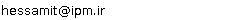••••••••## “Tatiana Hessami Pilehrood”Tel:  (+98)(381)4424419
Fax:  (+98)(381)4424419
Email:### IPM Positions

Non Resident Researcher (non-resident), School of Mathematics
(2010 - 2012 )

### Past IPM Positions

Associate Researcher (non-resident), School of Mathematics
(2006 - 2009)
Associate Researcher (non-resident), School of Mathematics
(2004 - 2006)

Associate Researcher (non-resident), School of Mathematics
(2002 - 2003)

### Non IPM Affiliations

Associate Professor of Shahrekord University

### Research Interests

Transcendental Number Theory, Diophantine Approximations, Linear Independence and Irrationality of Numbers, Hypergeometric Functions.

### Present Research Project at IPM

Research program

Problem 1. The generalized polylogarithmic function is defined by the series

 Ls(a, z)= ? ? n=0 zn (n+a)s ,

where s ? N, a ? Q, 0 < a ? 1, |z| ? 1 for s ? 2 and |z| < 1 for s=1.

Note that polylogarithmic function is connected with the many remarkable constants such as z(2k+1)=L2k+1(1,1) (Riemann zeta function values at odd points, k ? 2),

 G=1/4?L2(1/2, -1) (Catalan?s constant),

 ? ? n=1 1 n2 2n =L2(1, 1/2)=p2/12-1/2(log2)2

which are not yet known to be irrational.

Almost all known results on the values of polylogarithms Ls(a, z) are proved for rational points close to zero. It is an interesting but very difficult problem to study the values at points that are far from zero, for example, on the boundary of the disc of convergence. In this connection we note Apery''s theorem (1978) that L3(1, 1)=z(3) is irrational, recent Rivoal''s theorem (2000) that there exist infinitely many irrational numbers among z(3), z(5), z(7), ?; Zudilin''s result (2001) that at least one of the four numbers

 z(5),z(7), z(9), z(11)

is irrational, and also Hata''s theorem (1993) on the values of dilogarithm that L2(1, 1/q) is irrational for any integer q ? (-?, -5]?[7, ?), and author''s result (1999) that at least one of the numbers L3(1, 1/q), L2(1, 1/q) is irrational for any integer q ? (-?, -1]?[3, ?).

Problem 2. Obtaining lower bounds for linear forms in values of generalized hypergeometric functions that depend on all of the coefficients. Particular cases of this problem were considered early by author.

Problem 3. Diophantine equations.

### Related Papers

 1. Bivariate identities for values of the Hurwitz zeta function and supercongruencesElectron. J. Combin. 18 (2012), #P35  [abstract]
 2. On a continued fraction expansion for Euler's constantJ. Number Theory  (Accepted) [abstract]
 3. Rational approximations for values of Bell polynomials at points involving Euler s constant and zeta valuesAustral. Math. Soc. (Accepted) [abstract]
 4. Rational approximations for values of the digamma function and a denominators conjectureMath. Notes (Accepted) [abstract]
 5. A q-analogue of the bailey-Borwein-Bradley identityJournal of Symbolic Computation 46 (2011), 699-711  [abstract]
 6. Vacca-type series for values of the generalized Euler constant function and its derivativeJournal of Integer Sequences 13 (2010), 10.7.3  [abstract]
 7. Rational approximations for the quotient of gamma valuesIndag. Math. (N.S.) 20 (2009), 583-601  [abstract]
 8. An Apery-like continued fraction for πcothπJ. Difference Equ. Appl. (Accepted) [abstract]
 9. Generating function identities for ζ(2n+2), zeta(2n+3) via the WZ methodElectron. J. Combin. 15 (2008), 1-9  [abstract]
 10. On a conjecture of ErdosMath. Notes 83 (2008), 281-284  [abstract]
 11. Infinite sums as linear combinations of polygamma functionsActa Arith. 130 (2007), 231-254  [abstract]
 12. Apéry-like recursion and continued fraction for π coth π ( In: Diophantische approximationen)[abstract]
 13. On conditional irrationality measures for values of the digamma fuctionJ. Number Theory 123 (2007), 241-253  [abstract]
 14. Arithmetical properties of some series with logarithmic coefficientMath. Z. 255 (2007), 117-131  [abstract]
 15. Irrationality of the sums of zeta valuesMath. Notes 79 (2006), 607-618  [abstract]
 16. On sums of two rational cubesIndian J. Pure Appl. Math. 36 (2006), 707-717  [abstract]
 17. Irrationality of certain numbers that contain values of the di- and trilogarithmMath. Z. 254 (2006), 299-313  [abstract]
 18. On the diophantine equation x2+3=pynIndian J. Pure Appl. Math. 36 (2005), 431-439  [abstract]
 19. Lower Bounds for linear forms in values of polylogarithmsMath. Notes 77 (2005), 573-579  [abstract]
 20. Criteria for irrationality of generalized Euler's constantJ. Number Theory 108 (2004), 169-185  [abstract]
 [Back]
scroll left or right